### MODEL Statement

MODEL dependent-variables = independent-effects </ options> ;

The MODEL statement names the dependent variables and independent effects. The syntax of effects is described in the section Specification of Effects. For any model effect involving classification variables (interactions as well as main effects), the number of levels cannot exceed 32,767. If no independent effects are specified, only an intercept term is fit. You can specify only one MODEL statement (in contrast to the REG procedure, for example, which allows several MODEL statements in the same PROC REG run).

Table 44.9 summarizes the options available in the MODEL statement.

Table 44.9: MODEL Statement Options

Option

Description

Produce effect size information

Adds measures of effect size to each analysis of variance table

Produce tests for the intercept

Produces the hypothesis tests associated with the intercept

Omit the intercept parameter from model

Omits the intercept parameter from the model

Produce parameter estimates

Produces parameter estimates

Produce tolerance analysis

Displays the tolerances used in the SWEEP routine

Suppress univariate tests and output

Suppresses the display of univariate statistics

Display estimable functions

Displays the general form of all estimable functions

Displays the Type I estimable functions and computes sums of squares

Displays the Type II estimable functions and computes sums of squares

Displays the Type III estimable functions and computes sums of squares

Displays the Type IV estimable functions computes sums of squares

Specifies that the estimable functions should be displayed as an aliasing structure

Control hypothesis tests performed

Displays Type I sum of squares

Displays Type II sum of squares

Displays Type III sum of squares

Displays Type IV sum of squares

Produce confidence intervals

Specifies the level of significance

Produces confidence limits for individual predicted values

Produces confidence limits for a mean predicted value

Produces confidence limits for the parameter estimates

Display predicted and residual values

Displays observed, predicted, and residual values

Display intermediate calculations

Displays the augmented inverse (or generalized inverse)matrix

Displays the augmentedcrossproducts matrix

Tune sensitivity

Tunes the sensitivity of the regression routine to linear dependencies

Tunes the sensitivity of the check for estimability for Type III and Type IV functions

The options available in the MODEL statement are described in the following list.

ALIASING

specifies that the estimable functions should be displayed as an aliasing structure, for which each row says which linear combination of the parameters is estimated by each estimable function; also, this option adds a column of the same information to the table of parameter estimates, giving for each parameter the expected value of the estimate associated with that parameter. This option is most useful in fractional factorial experiments that can be analyzed without a CLASS statement.

ALPHA=p

specifies the level of significance p for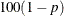% confidence intervals. By default, p is equal to the value of the ALPHA= option in the PROC GLM statement, or 0.05 if that option is not specified. You can use values between 0 and 1.

CLI

produces confidence limits for individual predicted values for each observation. The CLI option is ignored if the CLM option is also specified.

CLM

produces confidence limits for a mean predicted value for each observation.

CLPARM

produces confidence limits for the parameter estimates (if the SOLUTION option is also specified) and for the results of all ESTIMATE statements.

E

displays the general form of all estimable functions. This is useful for determining the order of parameters when you are writing CONTRAST and ESTIMATE statements.

E1

displays the Type I estimable functions for each effect in the model and computes the corresponding sums of squares.

E2

displays the Type II estimable functions for each effect in the model and computes the corresponding sums of squares.

E3

displays the Type III estimable functions for each effect in the model and computes the corresponding sums of squares.

E4

displays the Type IV estimable functions for each effect in the model and computes the corresponding sums of squares.

EFFECTSIZE

adds measures of effect size to each analysis of variance table displayed by the procedure, except for those displayed by the TEST option in the RANDOM statement, by CONTRAST and TEST statements with the E= option, and by MANOVA and REPEATED statements. The effect size measures include the intraclass correlation and both estimates and confidence intervals for the noncentrality for the F test, as well as for the semipartial and partial correlation ratios. For more information about the computation and interpretation of these measures, see the section Effect Size Measures for F Tests in GLM.

INTERCEPT
INT

produces the hypothesis tests associated with the intercept as an effect in the model. By default, the procedure includes the intercept in the model but does not display associated tests of hypotheses. Except for producing the uncorrected total sum of squares instead of the corrected total sum of squares, the INT option is ignored when you use an ABSORB statement.

INVERSE
I

displays the augmented inverse (or generalized inverse)matrix: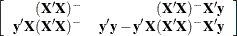The upper-left corner is the generalized inverse of, the upper-right corner is the parameter estimates, and the lower-right corner is the error sum of squares.

NOINT

omits the intercept parameter from the model. The NOINT option is ignored when you use an ABSORB statement.

NOUNI

suppresses the display of univariate statistics. You typically use the NOUNI option with a multivariate or repeated measures analysis of variance when you do not need the standard univariate results. The NOUNI option in a MODEL statement does not affect the univariate output produced by the REPEATED statement.

P

displays observed, predicted, and residual values for each observation that does not contain missing values for independent variables. The Durbin-Watson statistic is also displayed when the P option is specified. The PRESS statistic is also produced if either the CLM or CLI option is specified.

SINGULAR=number

tunes the sensitivity of the regression routine to linear dependencies in the design. If a diagonal pivot element is less than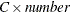as PROC GLM sweeps thematrix, the associated design column is declared to be linearly dependent with previous columns, and the associated parameter is zeroed.

The C value adjusts the check to the relative scale of the variable. The C value is equal to the corrected sum of squares for the variable, unless the corrected sum of squares is 0, in which case C is 1. If you specify the NOINT option but not the ABSORB statement, PROC GLM uses the uncorrected sum of squares instead.

The default value of the SINGULAR= option,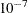, might be too small, but this value is necessary in order to handle the high-degree polynomials used in the literature to compare regression routines.

SOLUTION

produces a solution to the normal equations (parameter estimates). PROC GLM displays a solution by default when your model involves no classification variables, so you need this option only if you want to see the solution for models with classification effects.

SS1

displays the sum of squares associated with Type I estimable functions for each effect. These are also displayed by default.

SS2

displays the sum of squares associated with Type II estimable functions for each effect.

SS3

displays the sum of squares associated with Type III estimable functions for each effect. These are also displayed by default.

SS4

displays the sum of squares associated with Type IV estimable functions for each effect.

TOLERANCE

displays the tolerances used in the SWEEP routine. The tolerances are of the form C/USS or C/CSS, as described in the discussion of the SINGULAR= option. The tolerance value for the intercept is not divided by its uncorrected sum of squares.

XPX

displays the augmentedcrossproducts matrix: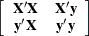ZETA=value

tunes the sensitivity of the check for estimability for Type III and Type IV functions. Any element in the estimable function basis with an absolute value less than the ZETA= option is set to zero. The default value for the ZETA= option is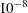.

Although it is possible to generate data for which this absolute check can be defeated, the check suffices in most practical examples. Additional research is needed in order to make this check relative rather than absolute.